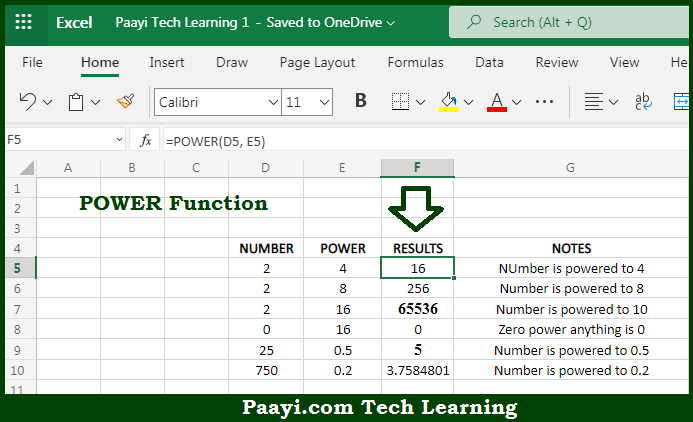# Learn How to Use Microsoft Excel POWER Function

Written by | 0 Comments | 418 Views

In this article, you will learn how to use the Microsoft Excel POWER function and its prime function in Microsoft Excel. You will also get to know the Microsoft Excel POWER function return value and syntax with the help of some examples.

Microsoft Excel POWER Function

The main function of the Microsoft Excel POWER function is to raise the number to the given power. That implies, with the help of the POWER function you can able to return the number according to the given power. It should be noted that the POWER function works like an exponent in the standard mathematics equation. So, with the help of the POWER function, you can able to get the number to the given power.

Return Value of POWER Function

The return value will be the number raised to the power.

Syntax of POWER Function

=POWER(number, power)

Where the arguments:

• number: This is the number you want to raise to a power.
• power: This is the power you want to raise a number (exponent).

## How to Use Microsoft Excel POWER Function?So we know that Microsoft Excel POWER function you can able to raise the number to the given power. That implies, with the help of the POWER function you can able to return the number according to the given power. It should be noted that the POWER function works like an exponent in the standard mathematics equation. So, with the help of the POWER function, you can able to get the number to the given power.